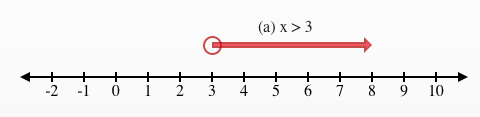### Home > CCAA > Chapter 6 Unit 4 > Lesson CC2: 6.1.3 > Problem6-29

6-29.

Graph each of the following inequalities on a number line.

When graphing an inequality on a number line, you should highlight all values that make the inequality true.

1. $x>3$

What values make the inequality $x>3$ true?

Possible values: $3.0000001$, $3.1$, $3.35$, $3.98$, $4$, $5$, $6$, $7$, etc.
Note: $3>3$ is not a true statement, so it is not on the list and should not be highlighted on the number line.

Highlight the values on a number line. Note that $3$ is not included, so it gets an unfilled circle.1. $x\le5$

1. $x\ge-4$